Question of The Day15-11-2019

A cone of height 16 cm into two parts by a line parallel to the base of the cone such that a smaller cone of height 4 cm and a frustum are obtained. What will be the ratio of the curved surface area of the frustum and the larger cone?

Correct Answer : c ) 15 : 16

Explanation :

Let us assume the height, radius and slant height of a smaller cone are h, r, and l respectively

Let us assume the height, radius and slant height of a larger cone are H, R and L respectively

Depicting the above information in the figure we get,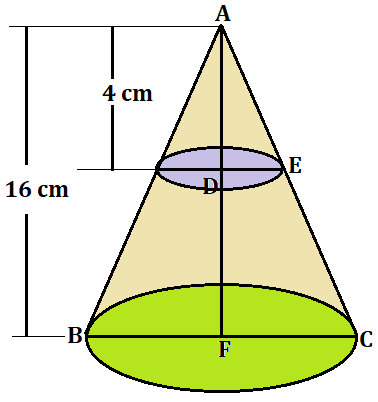From the above figure,

In triangle ADE and triangle AFC

∠DAE = ∠FAC (common angle)

Therefore from Angle – Angle (AA) property we can say that ▲ADC ≅ ▲AEC

Thus,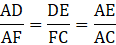Therefore,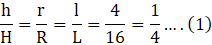As we know that

The curved surface area of the smaller cone (CSA)S = πrl ….. (2)

The curved surface area of larger cone (CSA)L = πRL …… (3)

Dividing equation 3 by 2 we get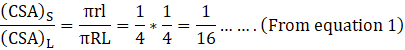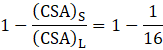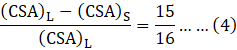As Curved surface area of Frustum (CSA)F will be = (CSA)L – (CSA)S ….. (5)

By comparing equation 4 and 5 we get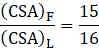Hence, (c) is the correct answer.

Such type of question is asked in exams like SSC CGL, SSC MTS, SSC CPO, SSC CHSL, RRB JE, RRB NTPC, RRB GROUP D, RRB OFFICER SCALE-I, IBPS PO, IBPS SO, RRB Office Assistant, IBPS Clerk, RBI Assistant, IBPS RRB OFFICER SCALE 2&3, etc. Try and attempt free mock tests at PendulumEdu. Read Daily Current Affairs and Banking Awareness to improve your preparation level for the government exams.0